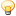1. ##Grade system in the US

I know that the US use five point system in schools: ABCDF (without E). But I'm wondering how they calculate an average grade at the end of the year? Do they convert letters into numbers, then calculate average grade and convert it back? Why bother? Using numeric grades are much easier.Reply With Quote

2. Excellent question. Let me explain it in three parts.

The first part is yes. Your ABCDE (we have E's here) are converted into a number.
A=4
B=3
C=2
D=1
E=0

The grade point average (GPA) is the average number of grade points earned per course in Grades 9 through 12, including grade points earned for successful completion of certain high school level courses taken while in middle school. The GPA is computed by dividing the total number of grade points earned by the total number of course credits included in the computation. The GPA should be rounded to two decimal points by rounding the second decimal position upward if the third decimal position is greater than four.

Now comes part 2 of the explanation:

Most schools offer both on-level and "Honors courses"; however, honors courses require greater use of abstract and higher-level thinking skills, and require research and projects of a more rigorous and challenging nature. So, since an Honors class is harder, they give you a bump up in the number of points that you get in your grade or a "weighted grade point average":

Weighted grade points are similar to grade points except that weighted grade points differentiate between regular courses and honors courses, and all courses designated advanced level courses. Weighted grade points are the numeric equivalent of a student’s grade in a credit-bearing course according to the following scale:

A = 5
B = 4
C = 3
D = 1
E = 0

The weighted grade point average (WGPA) is a recalculation of the GPA substituting weighted grade points for regular grade points earned in courses designated as honors or advanced level. The WGPA is computed by dividing the total number of grade points earned by the total number of course credits included in the computation. The WGPA should be rounded to two decimal points by
rounding the second decimal position upward if the third decimal position is greater than four.

Some school districts also will give grades of "+" or "-" and then give a 3.5 or 2.5 numeric scores accordingly. Our school system does not do that.

Examples:
ABA = A
ABB = B
ABC = B
ABD = B
ABE = B
CBA = B
CBB = B
CBC = C
CBD = C
CBE = C

Now the problem comes in with these honor classes. Not all colleges want a weighted GPA and it's not fair to a student who is taking a college level class in 9th grade or high school level class in 7th grade who gets a B or a C that their GPA is now in the dumpster. So, to help them out, we have the actual grades listed to say, "Hey, I earned a B in Algebra in 7th grade, give me a break. Look at my transcript."Reply With Quote

####Posting Permissions

• You may not post new threads
• You may not post replies
• You may not post attachments
• You may not edit your posts
•